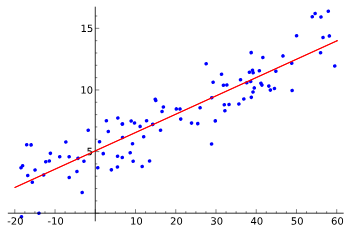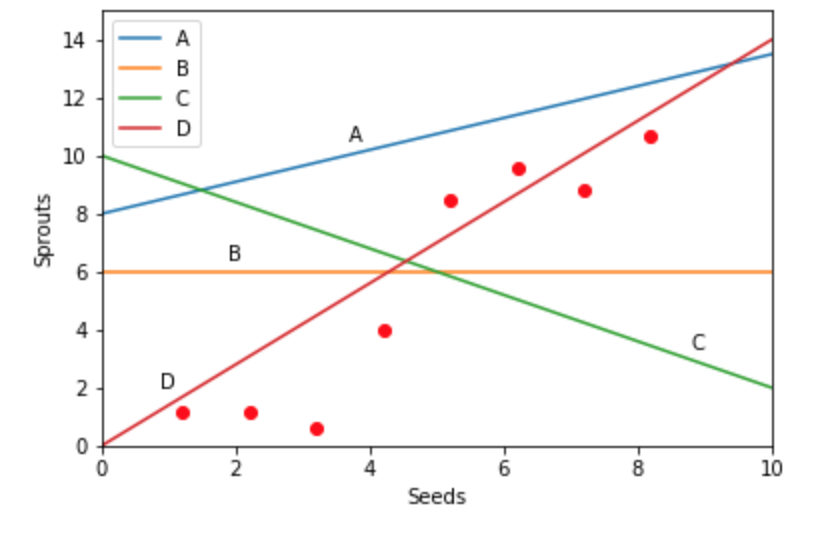### Machine Learning

Many data sets have an approximately linear relationship between variables. In these cases, we can predict one variable using a known value for another using a best-fit line, a line of the form $y = mx+b$ that follows the trends in the data as closely as possible.Here, $x$ is called the predictor variable because it will be used to predict $y,$ while $y$ is often called the response variable.

This technique is known as linear regression, and although it is one of the simplest machine learning techniques, it is often surprisingly powerful.

# Introduction to Linear Regression

Of course, linear regression isn’t limited to just one predictor variable. The key concept behind it is the idea that a change in one or more predictor variables will produce a linear change in the response variable.

We can think of this as a way to estimate the response variable by summing many weighted predictor variables, each of which has an impact on the final guess proportional to its importance.

For now, we'll limit ourselves to a single predictor variable in order to learn about linear regression more intuitively.

# Introduction to Linear Regression

For ten years, Alfred has been planting trees in his exceedingly large backyard. Each year, he records how many seeds he planted in the spring, as well as how many new sprouts there are by fall.

With the data points graphed below, which line best represents the relationship between seeds planted and sprouts observed? Use your intuition!×